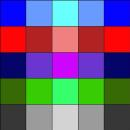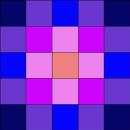### Submit a Resource

NRICH: Attractive Tablecloths

Charlie has been designing tablecloths for each weekday. He likes to use as many colours as he possibly can but insists that his tablecloths have some symmetry.

The 5 by 5 tablecloths below each satisfy a different symmetry rule.Monday’s 5 by 5 tablecloth has just 1 line of symmetry. Design some tablecloths of other sizes with just 1 line of symmetry. Check you agree that a 7 by 7 tablecloth needs 28 colours. Can you find a way of working out how many colours would be needed for an n by n tablecloth (where n is odd)?Tuesday’s 5 by 5 tablecloth has rotational symmetry of order 4, and no lines of symmetry. Design some tablecloths of other sizes with rotational symmetry of order 4, and no lines of symmetry. Check you agree that a 7 by 7 tablecloth needs 13 colours. Can you find a way of working out how many colours would be needed for an n by n tablecloth (where n is odd)?Wednesday’s 5 by 5 tablecloth has 2 lines of symmetry (horizontal and vertical), and rotational symmetry of order 2. Design some tablecloths of other sizes with 2 lines of symmetry, and rotational symmetry of order 2. Check you agree that a 7 by 7 tablecloth needs 16 colours. Can you find a way of working out how many colours would be needed for an n by n tablecloth (where n is odd)?Thursday’s 5 by 5 tablecloth has 2 (diagonal) lines of symmetry and rotational symmetry of order 2. Design some tablecloths of other sizes with 2 (diagonal) lines of symmetry and rotational symmetry of order 2. Check you agree that a 7 by 7 tablecloth needs 16 colours. Can you find a way of working out how many colours would be needed for an n by n tablecloth (where n is odd)?Friday’s 5 by 5 tablecloth has 4 lines of symmetry and rotational symmetry of order 4. Design some tablecloths of other sizes with 4 lines of symmetry and rotational symmetry of order 4. Check you agree that a 7 by 7 tablecloth needs 10 colours. Can you find a way of working out how many colours would be needed for an n by n tablecloth (where n is odd)?

EXTENSION
At weekends Charlie likes to use tablecloths with an even number of squares. Investigate the number of colours that are needed for different types of symmetric n by n tablecloths where n is even.

##### Age 14 to 16

Math Topics
Algebra & Pre-Algebra, Geometry, Symmetry
High School

## Organization

NRICH (University of Cambridge)

Board/Card Game
Tutorial